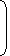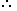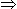# Tricks to Profit and Loss Problems for Competitive Exams##### IMPORTANT FACTS

Cost Price:

The price, at which an article is purchased, is called its cost price, abbreviated as C.P.

selling price:

The price, at which an article is sold, is called its selling prices, abbreviated as S.P.

Profit or Gain:

If S.P. is greater than C.P., the seller is said to have a profit or gain.

Loss:

If S.P. is less than C.P., the seller is said to have incurred a loss.

IMPORTANT FORMULAE:

1.In case of profit,

profit = selling price – cost price

selling price = cost price + profit

cost price = selling price – profit

2.In case of loss,

loss = cost price – selling price

selling price = cost price – loss

cost price = selling price + loss

3. Profit percentage and loss percentage

Profit percentage and loss percentage are always calculated on cost price unless otherwise stated.

In case of profit,

profit percentage=profit×100cost priceprofit percentage=profit×100cost price

selling price=cost price+cost price×profit percentage100=cost price(100+profit percentage)100selling price=cost price+cost price×profit percentage100=cost price(100+profit percentage)100

cost price=100×selling price100+profit percentagecost price=100×selling price100+profit percentage

Example: If an object is sold at a profit of 20%,20%,
selling price =120%=120% of cost price

In case of loss,

loss percentage=loss×100cost priceloss percentage=loss×100cost price

selling price=cost pricecost price×loss percentage100=cost price(100loss percentage)100selling price=cost price−cost price×loss percentage100=cost price(100−loss percentage)100

cost price=100×selling price100loss percentagecost price=100×selling price100−loss percentage

Example: If an object is sold at a loss of 20%20%
selling price =80%=80% of cost price

4. Selling at same price

(4.1) Suppose a person sells two objects at the same price, one at a profit of x1%x1% and another at a profit of x2%.x2%. Then,
net profit percentage =100(x1+x2)+2x1x2200+x1+x2=100(x1+x2)+2x1x2200+x1+x2
Note:
(a) for loss, use -sign for x1x1 and/or x2x2 as applicable.
(b) If the formula evaluates to a -ve value, it means there is a net loss
EXAMPLES ON PROFIT AND LOSS
1. Alfred buys an old scooter for Rs. 4700 and spends Rs. 800 on its repairs. If he sells the scooter for Rs. 5800, his gain percent is:
A.
 4 4 % 7
B.
 5 5 % 11
C.
 10%
D 12%

Explanation:

Cost Price (C.P.) = Rs. (4700 + 800) = Rs. 5500.

Selling Price (S.P.) = Rs. 5800.

Gain = (S.P.) – (C.P.) = Rs.(5800 – 5500) = Rs. 300.

 Gain % =300 x 100% = 5 5 % 5500 11

2.The cost price of 20 articles is the same as the selling price of x articles. If the profit is 25%, then the value of x is:

 A. 15 B. 16 C. 18 D. 25

Explanation:

Let C.P. of each article be Re. 1 C.P. of x articles = Rs. x.

S.P. of x articles = Rs. 20.

Profit = Rs. (20 – x).20 – x x 100 = 25x2000 – 100x = 25x

125x = 2000x = 16.

Correct! Wrong!

Correct! Wrong!

Correct! Wrong!

Correct! Wrong!

#### 5.Sam purchased 20 dozens of toys at the rate of Rs. 375 per dozen. He sold each one of them at the rate of Rs. 33. What was his percentage profit?

Correct! Wrong!

PROFIT AND LOSS QUIZ
hurrah!!! You have done it..